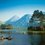# Can you find out what's wrong ?

I'll present a proof that $$0 = 1$$. Of-course there is an error in the proof. Can you find it?

## $\large \textbf{Proof}$

Let us start with a vector field $\overrightarrow{v} = \dfrac{1}{r^2} \hat{r}$.

Let us find the Divergence of this field. $\overrightarrow{\nabla} . \overrightarrow{v} = \frac{1}{r^2} \frac{\partial}{\partial r} \left( r^2 \dfrac{1}{r^2} \right) = \boxed{0}$

Now let us calculate the flux of this field. $\oint_{S}{ \overrightarrow{v}. \overrightarrow{da}} = 4\pi$

Green’s Theorem state that $\int_{V} {\left (\overrightarrow{\nabla} . \overrightarrow{v} \right ) d\tau} = \oint_{S}{ \overrightarrow{v}. \overrightarrow{da}}$

Applying it we see L.H.S = 0 since $\overrightarrow{\nabla} . \overrightarrow{v} = 0$ and R.H.S = $4\pi$.

This implies $0 = 4\pi$.
Dividing by $4\pi$ we get
$\boxed{ 0 = 1 }$

Hence proved.Note by Rajdeep Dhingra
5 years, 5 months ago

This discussion board is a place to discuss our Daily Challenges and the math and science related to those challenges. Explanations are more than just a solution — they should explain the steps and thinking strategies that you used to obtain the solution. Comments should further the discussion of math and science.

When posting on Brilliant:

• Use the emojis to react to an explanation, whether you're congratulating a job well done , or just really confused .
• Ask specific questions about the challenge or the steps in somebody's explanation. Well-posed questions can add a lot to the discussion, but posting "I don't understand!" doesn't help anyone.
• Try to contribute something new to the discussion, whether it is an extension, generalization or other idea related to the challenge.

MarkdownAppears as
*italics* or _italics_ italics
**bold** or __bold__ bold
- bulleted- list
• bulleted
• list
1. numbered2. list
1. numbered
2. list
Note: you must add a full line of space before and after lists for them to show up correctly
paragraph 1paragraph 2

paragraph 1

paragraph 2

[example link](https://brilliant.org)example link
> This is a quote
This is a quote
    # I indented these lines
# 4 spaces, and now they show
# up as a code block.

print "hello world"
# I indented these lines
# 4 spaces, and now they show
# up as a code block.

print "hello world"
MathAppears as
Remember to wrap math in $$ ... $$ or $ ... $ to ensure proper formatting.
2 \times 3 $2 \times 3$
2^{34} $2^{34}$
a_{i-1} $a_{i-1}$
\frac{2}{3} $\frac{2}{3}$
\sqrt{2} $\sqrt{2}$
\sum_{i=1}^3 $\sum_{i=1}^3$
\sin \theta $\sin \theta$
\boxed{123} $\boxed{123}$

Sort by:

@Rajdeep Dhingra is nishant abhangi better than u??

- 4 years, 4 months ago

Green's theorem does not hold as the divergence is not continuous inside a ball containing the origin.

- 5 years, 5 months ago

Yes , correct. To correct this error we have to use a Diract Delta Function.

- 4 years, 4 months ago

Hint 1: There is some thing fishy with the divergence.

- 5 years, 5 months ago